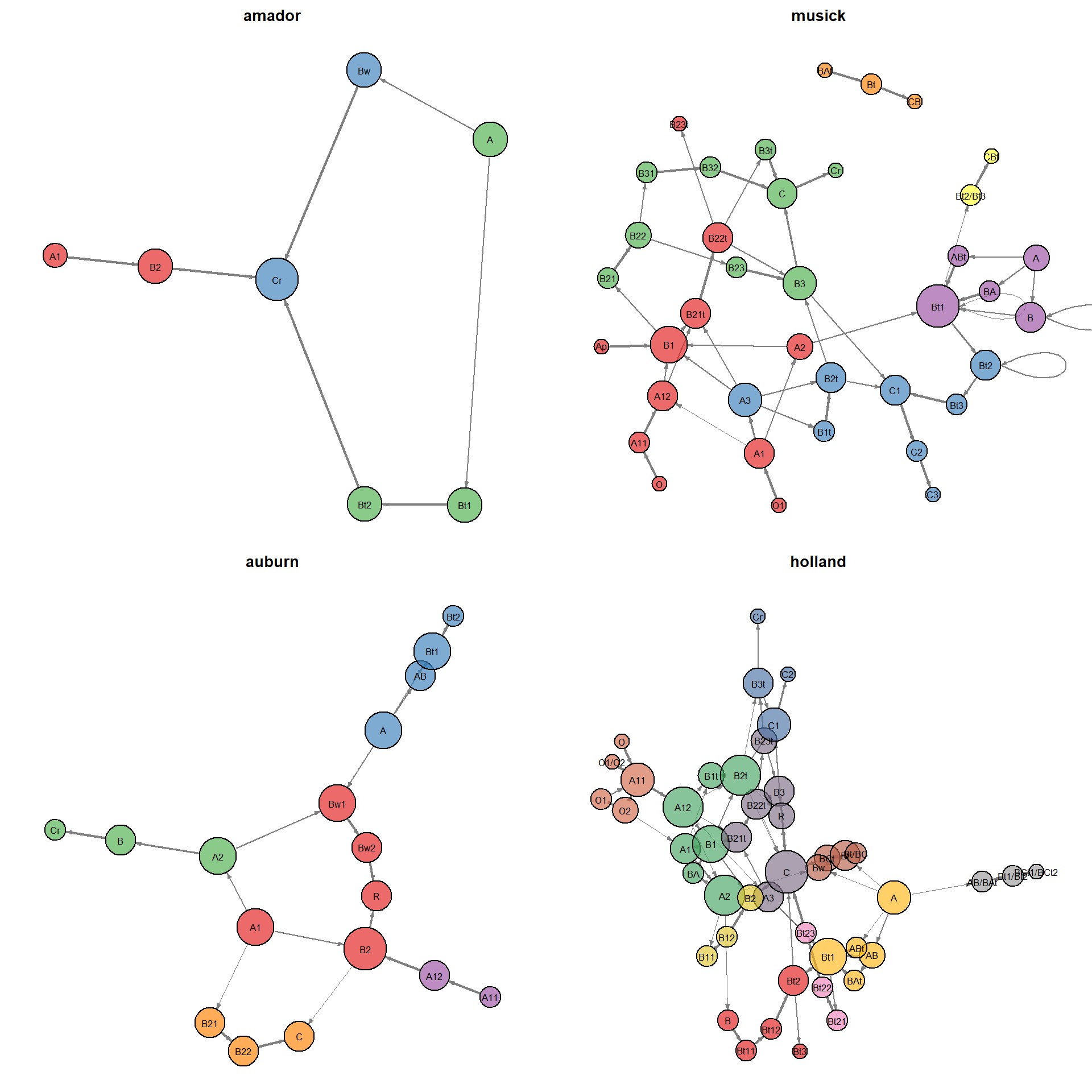# Horizon Sequence Transition Probability

D.E. Beaudette
2020-01-07
This document is based on `aqp` version 1.18.4 and `sharpshootR` version 1.5.3.

# Setup R Envionment

If you have never used the aqp or soildb packages before, you will likely need to install them. This only needs to be done once.

``````# stable version from CRAN + dependencies
install.packages("aqp", dep = TRUE)
install.packages("soilDB", dep = TRUE)
install.packages("sharpshootR", dep = TRUE)
install.packages("markovchain", dep = TRUE)``````

Once you have all of the R packages on which this document depends, it is a good idea to load them. R packages must be installed anytime you change versions of R (e.g., after an upgrade) and loaded anytime you want to access functions from within those packages.

``````library(markovchain)
library(aqp)
library(sharpshootR)
library(soilDB)
library(igraph)``````

# Sample Data

While the methods outlined in this document can be applied to any collection of pedons, it is convenient to work with a standardized set of data. You can follow along with the analysis by copying code from the following blocks and running it in your R session. The sample data used in this document is based on soil profiles that have been correlated to the Loafercreek soil series from the Sierra Nevada Foothill Region of California. Note that the internal structure of the `loafercreek` data is identical to the structure returned by `fetchNASIS()` from the soilDB package. All horizon-level values are pulled from the pedon horizon table of the pedons being analyzed.

``````# load sample data from the soilDB package
data(loafercreek, package = "soilDB")
pedons <- loafercreek
# plot profile sketches
par(mar = c(0, 0, 0, 0))
plot(pedons, name = "", print.id = FALSE, cex.names = 0.75, axis.line.offset = -4)``````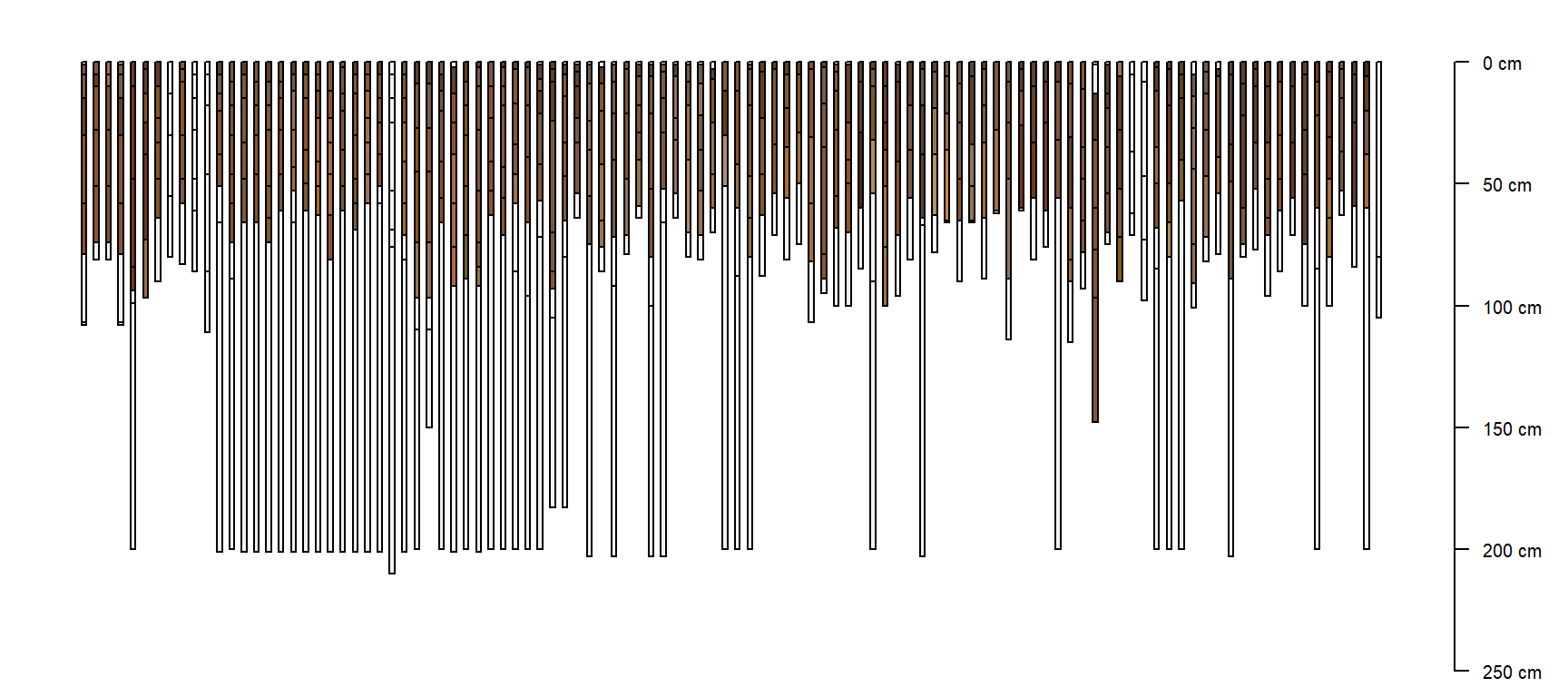Pedons correlated to the Loafercreek soil series.

The following code block demonstrates how to pull data in using the `fetchNASIS()` function from the soilDB package.

``````# first load the desired data set within NASIS into your NASIS selected set then load data from the
# NASIS selected set into R note that the `nullFragsAreZero` argument converts NULL rock fragment
# class data into 0s
pedons <- fetchNASIS(nullFragsAreZero = TRUE)
# optionally subset the data by taxon name - enter your taxon name
pedons <- pedons[grep(pattern = "ENTER_YOUR_TAXON_NAME", pedons\$taxonname, ignore.case = TRUE), ]``````

# Methods

``sort(table(pedons\$hzname), decreasing = TRUE)``
``````##
##    A  Bt1  Bt2   Cr  Bt3    R   BA   Oi  Crt  BCt   Bw 2Bt3  BAt   Bt  Bt4 2Bt2   A1   A2 2Bt4    C
##   97   94   89   49   47   41   31   24   20   16   10    8    8    8    8    7    7    7    6    6
##  CBt   2R   BC  2Cr 2Bt1 2Crt  ABt  Bw1  Bw2 2BCt 2Bt5  2CB 2CBt   AB   Ad   Ap    B  Bw3   Cd Cr/R
##    6    5    5    4    2    2    2    2    2    1    1    1    1    1    1    1    1    1    1    1
##   H1   Rt
##    1    1``````

Constructing a graph of transitions from one horizon to the next ("transition probabilities") can highlight those horizon designations that are most commonly used together. A follow-up tutorial on transition probability matrix interpretation is planned.

``````# sample data
data(sp4)
depths(sp4) <- id ~ top + bottom

# horizon transition probabilities: row -> col transitions
(tp <- hzTransitionProbabilities(sp4, "name"))``````
``````##     A A1 A2 AB       ABt        Bt       Bt1 Bt2 Bt3
## A   0  0  0  0 0.1111111 0.4444444 0.4444444   0   0
## A1  0  0  1  0 0.0000000 0.0000000 0.0000000   0   0
## A2  0  0  0  1 0.0000000 0.0000000 0.0000000   0   0
## AB  0  0  0  0 0.0000000 0.0000000 1.0000000   0   0
## ABt 0  0  0  0 0.0000000 0.0000000 1.0000000   0   0
## Bt  0  0  0  0 0.0000000 0.0000000 0.0000000   0   0
## Bt1 0  0  0  0 0.0000000 0.0000000 0.0000000   1   0
## Bt2 0  0  0  0 0.0000000 0.0000000 0.0000000   0   1
## Bt3 0  0  0  0 0.0000000 0.0000000 0.0000000   0   0
## attr(,"ties")
##  TRUE``````
``````# abuse sharpshootR code to display as graph
par(mar = c(0, 0, 0, 0), mfcol = c(1, 2))
plot(sp4)
plotSoilRelationGraph(tp, graph.mode = "directed", edge.arrow.size = 0.5, edge.scaling.factor = 2, vertex.label.cex = 0.75,
vertex.label.family = "sans")``````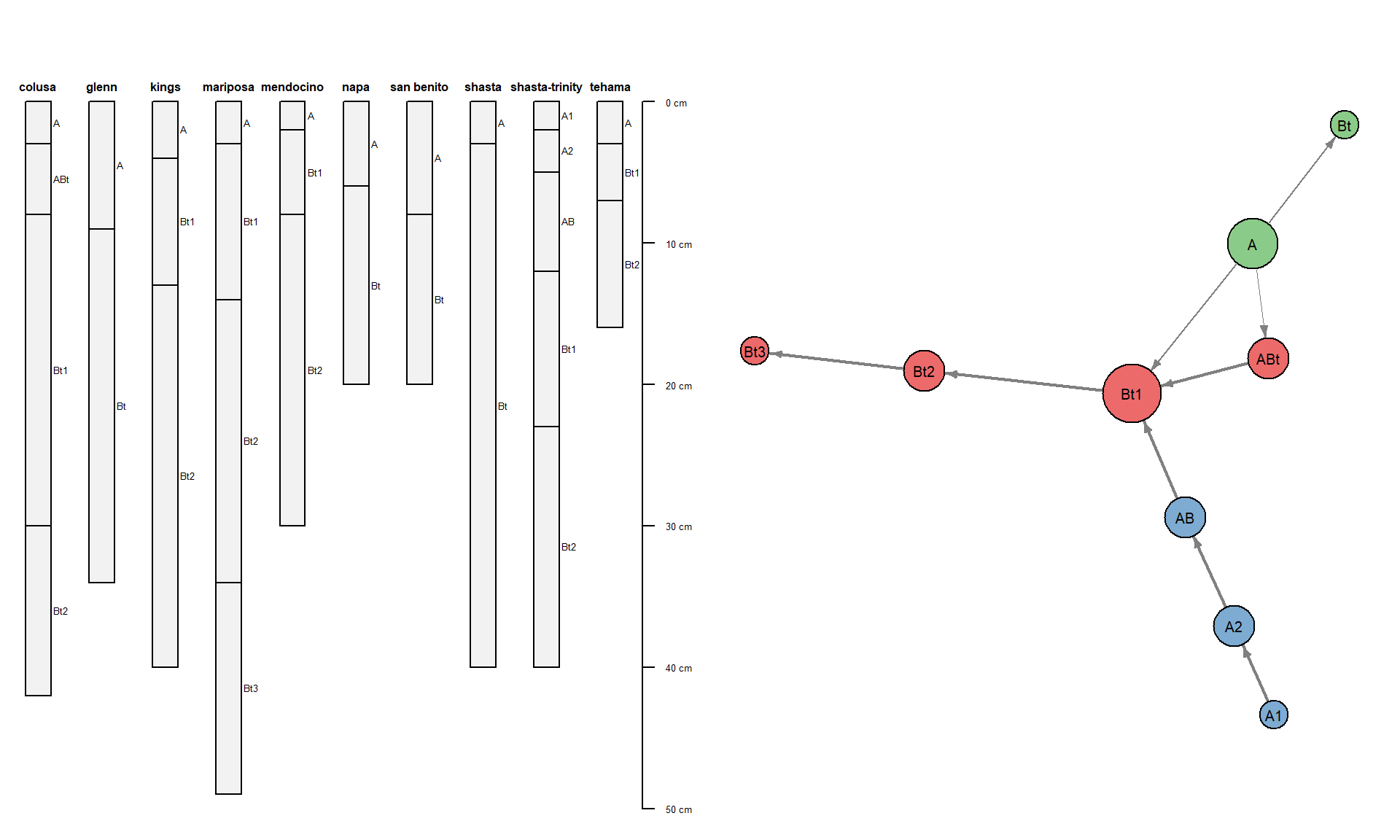Graph constructed from transition probabilities. Thicker lines denote more likely transitions.

More examples.

``````# try some other examples, seems logical
tp <- hzTransitionProbabilities(pedons, "hzname")
# tp <- hzTransitionProbabilities(pedons, 'genhz')

# abuse sharpshootR code sometimes default layout doesn't look so good
par(mar = c(0, 0, 0, 0), mfcol = c(1, 2))
plotSoilRelationGraph(tp, graph.mode = "directed", edge.arrow.size = 0.5)
plotSoilRelationGraph(tp, graph.mode = "directed", edge.arrow.size = 0.5, g.layout = layout_with_lgl)``````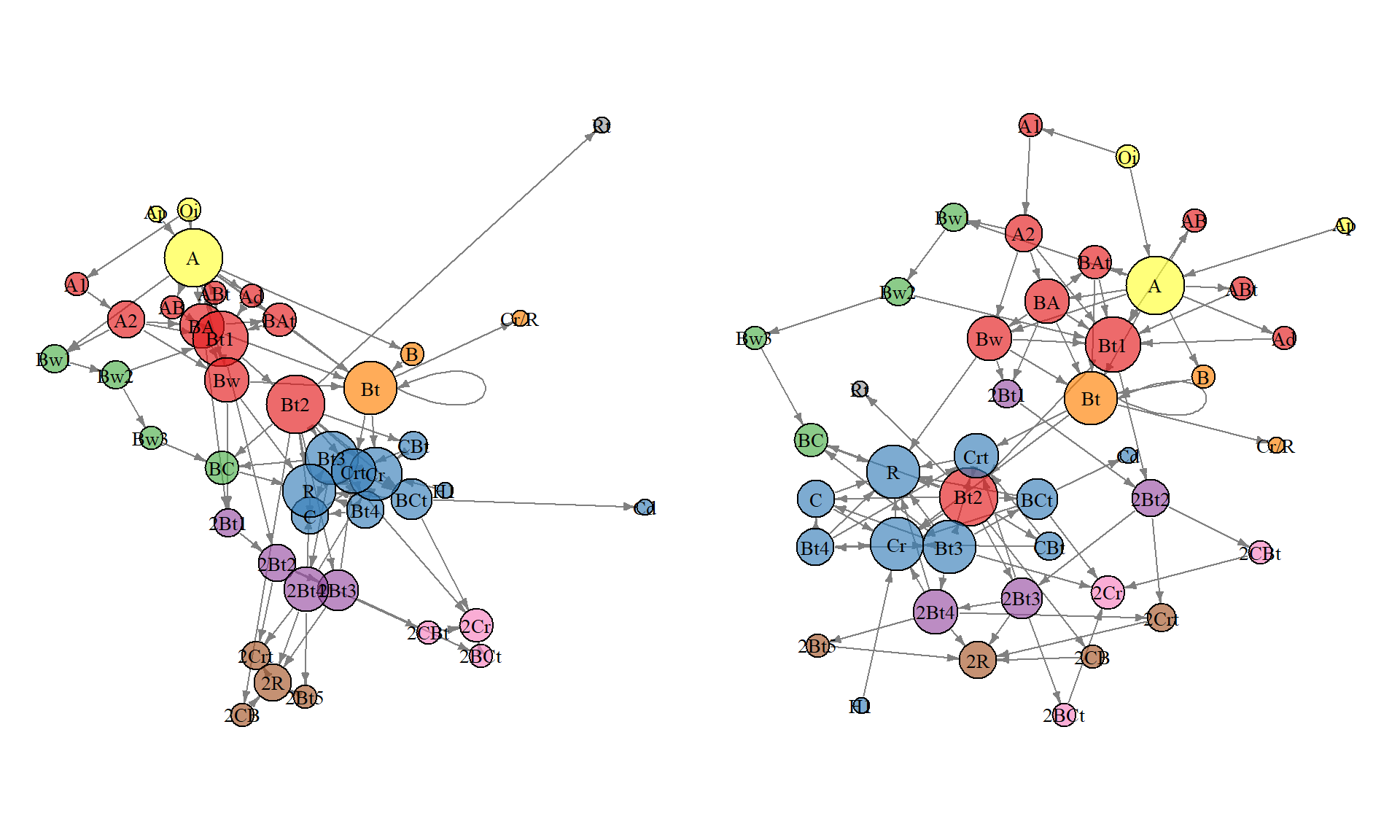Convert contingency table of generalized horizon labels to an adjacency (similar to transition probability) matrix.

``````tab <- table(pedons\$hzname, pedons\$genhz)

par(mar = c(0, 0, 0, 0), mfcol = c(1, 1))
plotSoilRelationGraph(m, graph.mode = "directed", edge.arrow.size = 0.5)``````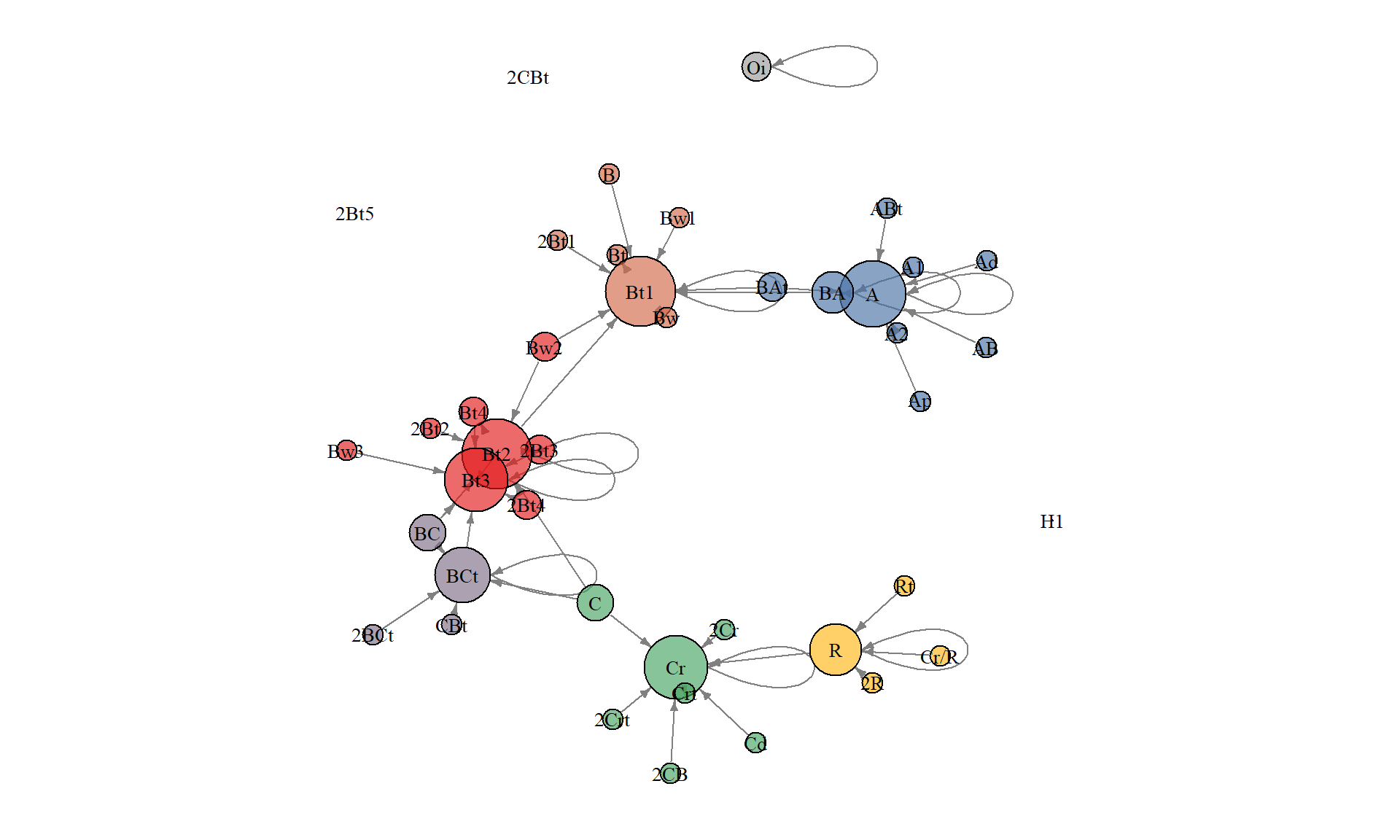Tinker with the markovchain package. Details here.

``````## init a markovchain object from TP this trquires 'looping' terminal states e.g. an absorbing MC:
## https://en.wikipedia.org/wiki/Absorbing_Markov_chain
tp.loops <- hzTransitionProbabilities(sp4, "name", loopTerminalStates = TRUE)

mc <- new("markovchain", states = dimnames(tp.loops)[], transitionMatrix = tp.loops)

# simple plot
plot(mc, edge.arrow.size = 0.5)``````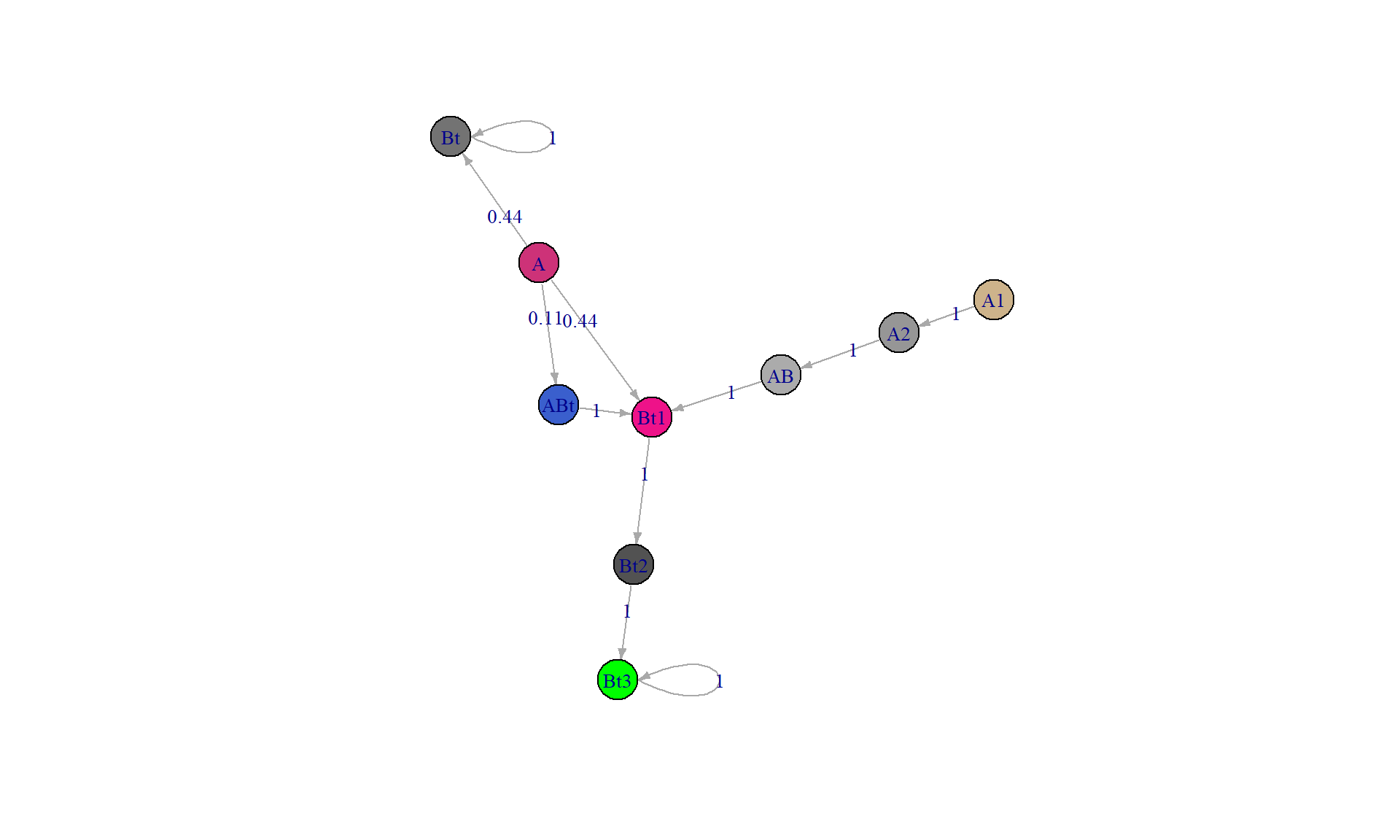``````# check absorbing states
absorbingStates(mc)``````
``##  "Bt"  "Bt3"``
``````# steady-state:
``````##      A A1 A2 AB ABt Bt Bt1 Bt2 Bt3
## [1,] 0  0  0  0   0  0   0   0   1
## [2,] 0  0  0  0   0  1   0   0   0``````
``````# try some more complex TP matrices
tp <- hzTransitionProbabilities(pedons, "hzname", loopTerminalStates = TRUE)
mc <- new("markovchain", states = dimnames(tp)[], transitionMatrix = tp)

# better plotting
par(mar = c(0, 0, 0, 0), mfcol = c(1, 2))
plot(mc, vertex.size = 10, edge.arrow.size = 0.5, edge.label.cex = 0.75, layout = layout_with_lgl)
plot(mc, vertex.size = 10, edge.arrow.size = 0.5, edge.label.cex = 0.75, layout = layout_as_tree)``````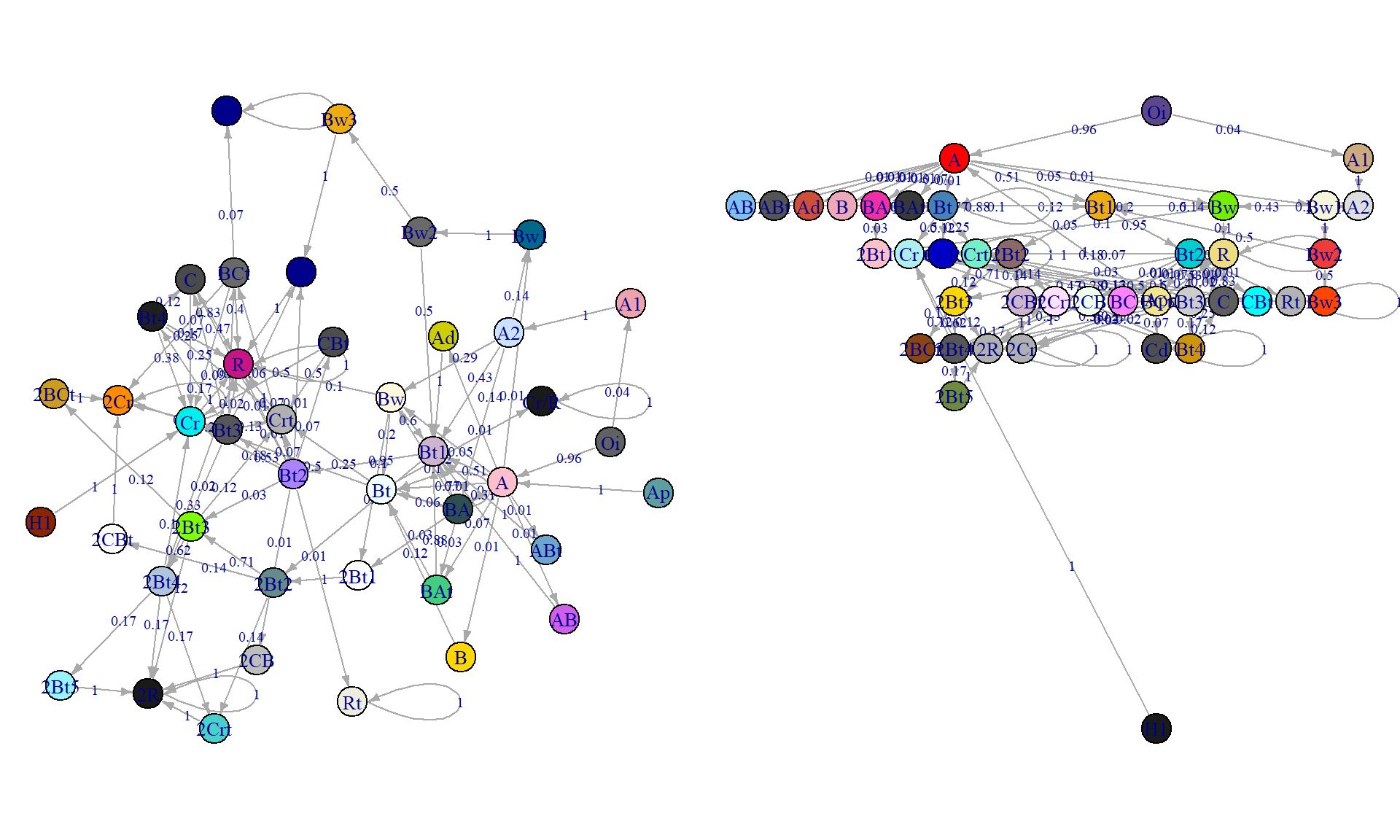``````# another
tp <- hzTransitionProbabilities(pedons, "genhz", loopTerminalStates = TRUE)
mc <- new("markovchain", states = dimnames(tp)[], transitionMatrix = tp)

# better plotting
par(mar = c(0, 0, 0, 0), mfcol = c(1, 2))
plot(mc, vertex.size = 10, edge.arrow.size = 0.5, edge.label.cex = 0.75, layout = layout_as_tree)
plot(mc, vertex.size = 10, edge.arrow.size = 0.5, edge.label.cex = 0.75, layout = layout_with_lgl)``````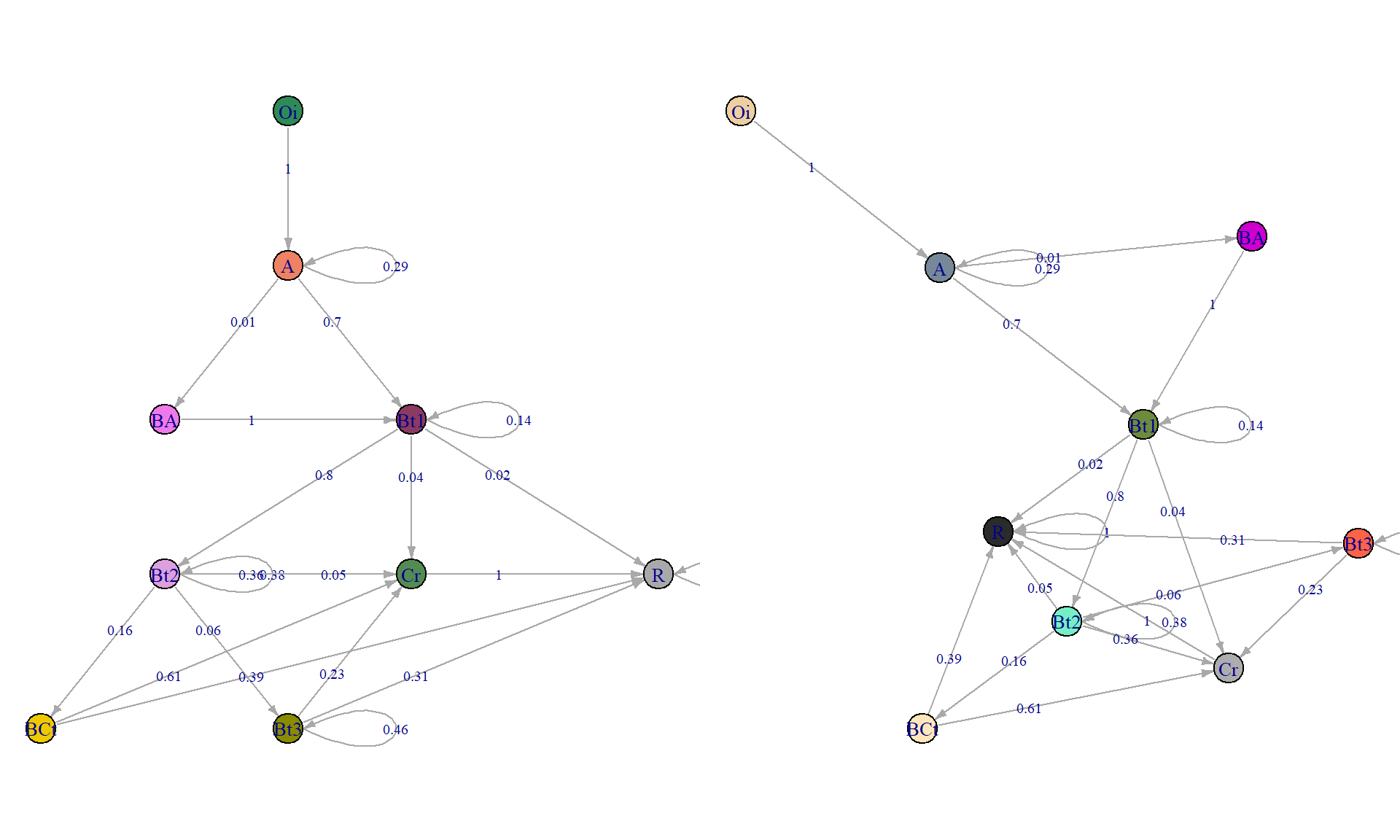Simulation from a markovchain object.

``````# simulate
rmarkovchain(10, mc, include.t0 = TRUE, t0 = "A")``````
``##   "A"   "A"   "Bt1" "Bt1" "Bt2" "Cr"  "R"   "R"   "R"   "R"   "R"``
``````# multiple simulations, as column vectors
replicate(10, rmarkovchain(10, mc, include.t0 = TRUE, t0 = "A"))``````
``````##       [,1]  [,2]  [,3]  [,4]  [,5]  [,6]  [,7]  [,8]  [,9]  [,10]
##  [1,] "A"   "A"   "A"   "A"   "A"   "A"   "A"   "A"   "A"   "A"
##  [2,] "Bt1" "Bt1" "Bt1" "Bt1" "Bt1" "Bt1" "Bt1" "Bt1" "A"   "Bt1"
##  [3,] "Bt2" "Bt2" "Bt2" "Bt2" "Bt2" "Bt2" "Bt2" "Bt2" "Bt1" "Bt2"
##  [4,] "Bt2" "Bt2" "Bt2" "Bt2" "Bt2" "Bt2" "BCt" "BCt" "Bt2" "BCt"
##  [5,] "Cr"  "Cr"  "Bt2" "BCt" "Bt2" "Bt2" "R"   "Cr"  "Cr"  "Cr"
##  [6,] "R"   "R"   "Cr"  "Cr"  "BCt" "Cr"  "R"   "R"   "R"   "R"
##  [7,] "R"   "R"   "R"   "R"   "R"   "R"   "R"   "R"   "R"   "R"
##  [8,] "R"   "R"   "R"   "R"   "R"   "R"   "R"   "R"   "R"   "R"
##  [9,] "R"   "R"   "R"   "R"   "R"   "R"   "R"   "R"   "R"   "R"
## [10,] "R"   "R"   "R"   "R"   "R"   "R"   "R"   "R"   "R"   "R"
## [11,] "R"   "R"   "R"   "R"   "R"   "R"   "R"   "R"   "R"   "R"``````
``````# what comes after a state?
conditionalDistribution(mc, "A")``````
``````##          A         BA        BCt        Bt1        Bt2        Bt3         Cr         Oi          R
## 0.29197080 0.00729927 0.00000000 0.70072993 0.00000000 0.00000000 0.00000000 0.00000000 0.00000000``````
``````# this is now in AQP, but requires markovchain package
mostLikelyHzSequence(mc, "A")``````
``##  "A"   "Bt1" "Bt2" "Cr"  "R"``

Try with some KSSL data.

``````# some series to query
s <- c("amador", "auburn", "musick", "holland")
s.data <- lapply(s, fetchKSSL)

par(mar = c(0, 0, 2, 0), mfcol = c(2, 2))
for (i in 1:length(s)) {
tp <- hzTransitionProbabilities(s.data[[i]], "hzn_desgn")
plotSoilRelationGraph(tp, graph.mode = "directed", edge.arrow.size = 0.3, edge.scaling.factor = 2,
main = s[i], vertex.label.cex = 0.75, vertex.label.family = "sans")
}``````